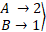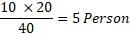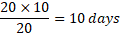• Save

# Time and work formulas with examples for SSC and Bank ExamsTime and work questions are important questions which usually asked in competitive exams. If you don't want to face any difficulty in time and work questions, you should know the proper way to use time and work formulas for ssc and bank exams.

Here in this blog, you can learn to use time and work formulas with different equations or examples. You can practice also time and work questions with answers to improve your performance.

## Time and work formulas for Competitive Exams:

What is Time and Work?

Performing or doing any involves efforts of person (s) over a period of time. Therefore, the number of person (P), the quantity of work (W) and the period of time taken (T) are important variables in problem-related to time and work.

Time (T) taken to do a work depends not only on how many persons are engaged to do it but also on how efficient they are. Efficiency here means rate of doing the work. This aspect comes into the picture, when the problem involves comparison of work being done by different categories of persons. For instance, efficiencies of man, woman boy.

Time period (T) taken to do work is usually given in number of days. But in many cases, in addition to the number of days, the problem also specifies the number of hours per day. The person when working in such cases, we use time (T) in border sense i.e., it includes not only the number of days for which the person works but also the number of hours per day that he or she works. Thus, time (T) is the product of number of days (say D) and working hours per day (say H) i.e., T = D x H.

### The problem on times and work can be solved by following two methods:

#### Ratio and Proportion Method:

Ex. A is twice efficient, then B if they complete a piece of work in 30 days working together, then in how many days A alone do the work?

(A)  20

(B) 35

(C) 45

(D) 90

Solution:3 × 30 = 90 days

Hence, the days taken by A to work alone is given by 90/2=45 days.

Ex. 10 Person can do a job in 20 days.

(i) How many persons are required to do the same job in 40 days?

(ii) In how many days 20 persons can complete the same job?

(A) 5, 10

(B) 6, 10

(C) 10, 10

(D) 5, 15

Solution:

(a) (i) To do the job in 20 days, we require 10 person.

∴ To do the job in 1 day, we require more

Number of person = 10 × 20 persons.

∴ To do the job in 40 days, we require less

Number of persons =(ii) 10 Persons do the job in 20 days

∴ 1 persons will do the job in more than 20 days = 20 × 10 days.

∴ 20 persons will do the job in less number of days =Some important facts are given below, go through it.

Important Facts of Time and work

1. If A can do a piece of work in n days, then A’s 1 days work = 1\n

2. If A’s 1 days work = 1\n, then A can finish the work in n days.

3. If A is thrice as good a workman as B, then :

Ratio of work done by A and B = 3 : 1.

Ratio of times taken by A and B to finish a work = 1 : 3.

Ask me in the comment section, if you face any problem while solving important Time and Work questions. Visit next page for more Time and Work problems.